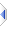# bigatom

arbitrary precision arithmetic, by Carlos G�mez Andreu (cargoan)

Depricated in favour of mpfr. True, this does not need dlls to be installed, but few end-user applications have much need for this sort of thing, and the performance difference speaks for itself. The one thing bigatom does better than mpfr is hold decimal fractions exactly, which has only proved significant so far for just one rosettacode task.
I don’t have any immediate plans to deliberately break or cease distributing this, you may be pleased to hear.
As a side note, I no longer have any plans to finish or properly document my builtins/bigint.e attempt, but likewise I’ll leave it where&how it is.

Included in the distribution as builtins\bigatom.e (not an autoinclude).

I (Pete Lomax) have translated the comments as best I can, and renamed the overrides log/exp/sqrt as b_a_log/b_a_exp/b_a_sqrt.
(Phix does not allow and in fact has recently been modified to expressly prohibit any builtin overides.)
Be advised that internally this uses simple digit-by-digit mechanisms and is not a high performance implementation.
(If it is possible that instead of digits 0..9 it used "digits" 0..999,999,999 then it might be ten times faster... Maybe...)

Once you understand how to create and display bigatoms, the rest is all pretty straightforward.

In the following, an object parameter indicates it can be atom/string/bigatom. Two things to remember are that you cannot store an atom/string in a bigatom, ie sometimes you must use bigatom res = ba_new(x) instead of res = x, and that all operations must be performed function-style, eg ba_add(a,b) rather than a+b.

bigatom - the bigatom type
sequence prev = ba_scale(object decs=-1, integer mode=-1) - set library precision and/or mode
bigatom ba = ba_new(object N) - create a bigatom instance -- N can be atom/string/bigatom
string s = ba_sprintf(sequence fmt, bigatom N) - get formatted string (%B) representation
ba_printf(integer file, sequence fmt, bigatom N) - print formatted string (%B) representation
string s = ba_sprint(bigatom N) - get standard string representation
ba_print(integer file, bigatom N) - print standard string representation
integer i = ba_scale_of(object N) -- the number of decimals before the decimal point, eg ba_scale_of(ba_new(123.4567)) is 3
integer i = ba_compare(object A, B) -- -1/0/1 (A<B/A=B/A>B) as per compare()
integer i = ba_sign(object N) -- -1/0/1 (A<0/A=0/A>0) as per sign()
bigatom ba = ba_add(object A, B)
bigatom ba = ba_sub(object A, B)
bigatom ba = ba_mul[tiply](object A, B, bool bRound=false)
bigatom ba = ba_div[ide](object A, B, bool bRound=false)
bigatom ba = ba_idiv[ide](object A, B)
bigatom ba = ba_remainder(object A, B) -- as per remainder()
bigatom ba = ba_mod(object A, B) -- as per mod() (aka a%b)
bigatom ba = ba_round(object N, atom precision=1, integer mode=0) -- rounding function (nb unlike round())
bigatom ba = ba_abs(object N) - absolute (unsigned) value, as per abs()
bigatom ba = ba_uminus(object N) - negated value, same result as ba := ba_sub(BA_ZERO,N) [but obviously less typing and slightly faster]
bigatom ba = ba_trunc(object N) - integer part of a bigatom, as per trunc()
bigatom ba = ba_frac(object N) - fractional part of a bigatom
bigatom ba = ba_floor(object N) - integer equal to or immediately less, as per floor()
bigatom ba = ba_ceil(object N) - integer equal to or immediately greater, as per ceil()
atom a = b_a_log(atom x) -- adapted from bigfixedmath.e, apparently better than log()
atom a = logb(atom x, atom base=10)
atom a = b_a_exp(atom x) -- adapted from library bc, apparently better than exp()
atom a = b_a_sqrt(atom x) -- apparently better than sqrt()
bigatom ba = ba_log(object N, bool bRound=false) -- the natural log, as per log()
bigatom ba = ba_exp(object N, bool bRound=false) -- returns E to the power of N
bigatom ba = ba_power(object N, exponent, bool bRound=false) -- as per power(x,exponent) (aka x^^exponent)
bigatom ba = ba_mod_exp(object base, exponent, modulus) -- as per ba_mod(ba_power(base,exponent,modulus)) (aka (b^^e)%m)
bigatom ba = ba_sqrt(object N, bool bRound=false) -- as per sqrt()
bigatom ba = ba_root(object N, exponent, bool bRound=false) -- returns x^(1/exponent)
bigatom ba = ba_log10(object N, bool bRound=false) -- as per log10()
bigatom ba = ba_logb(object N, base=10, bool bRound=false) -- log in any base
bigatom ba = ba_gcd(object m, n) -- as per gcd()
bigatom ba = ba_lcm(object m, n) -- as per lcm()
bigatom ba = ba_factorial(integer n) -- as per factorial()
atom a = bigatom_to_atom(bigatom N) -- an atom with the approximate value of a bigatom
object x = ba_euler(integer decs, integer output=0) -- e to decs places, as string(output=0) or bignum

The constants BA-ZERO and BA_ONE can be used in place of ba_new(0) and ba_new(1) respectively, in cases where 0 and 1 are not acceptable, and obviously using them may be noticeably faster than reconstructing them every time. Actually, there is now also a BA_TWO constant.